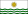Welcome to the geofictician wiki.

# Mahhalian Language

Mahhalian
Rakkan
Bodahhar (ቦጣኣር)
Pronunciation/bodaxat̚/
RegionOriginally Mahhal, now also some emigrant communities worldwide
Native speakers9-10 million (as of 2014)
Language familyHardan
Early formsProto-Mahhalian
• Classical Mahhalian
• Pre-modern Mahhalian
• Mahhalian
DialectsWest Rakkan (dominant)
Jessitimic
Oan
Puran
...many other dialects
Writing systemkastelly (Romantian script)
fidelly (Ghezic abugida)
hhenkully (native logographs)
Signed formsSigned Rakkan
Official status
Official language inTemplate:Collapsible list
OGFL`MHL`

The Mahhalian Language (native called Bodahhar "language of the sea") is the language, with all its dialects, used by the Mahhalian People in the Mahhalian Archipelago.

In fact, it is a collection of about 10 mutually comprehensible spoken dialects, unified by a single, archaic logographic writing system (much like Sinian). The writing system has been supplanted by the adopted Romantian alphabet and the Ghezic abugida in day-to-day usage, but no one graduates high school or is considered educated without mastering the 10,000+ characters of the native system. Typologically, the language is highly agglutinative and considered part of the Hardan language family, although this linkage is tenuous and some linguists have suggested relations with either the Algonkish languages or, less plausibly, the Altazorian language group. Because of the writing system, some have also suggested a relation with Ancient Sumopotamian, but this is not supported by the linguistic evidence except for the coincidental resemblance of the writing systems.

# Distribution

Currently there are upwards of 10 million speakers, worldwide. The following table shows distribution.

Country Number of speakers Notes
Template:Mahhal 9,000,000 based on 2012 federal census plus estimate
Template:Aŭstrasuno Tero 1,000,000Ardisphere 350,000 based on 2014 census
Template:Tárrases 92,600 based on 2010 census
Template:Gobrassanya 40,000
other... tbd

# Phonology

## Vowels

Mahhalian has eight cardinal vowels, shown at right in a standard IPA vowel chart. Positions of some vowels will vary by dialect, and in some archaic forms there are two additional vowels (written with digraphs "OY" and "AY", which are unrounded mid and low back vowels, /ɤ/ and /ɑ/).

## Consonants

The chart below pairs the consonant graphemes (in the "kastelly" writing system - see below) with their best IPA representation.

 Grapheme-IPA pairs chart for pulmonic consonants Place → Labial Coronal Dorsal Laryngeal ↓ Manner Bilabial Labio­dental Linguo­labial Dental Alveolar Palato-alveolar Retroflex Alveolo-palatal Palatal Velar Uvular Pharyngeal/ Epiglottal Glottal Nasal "mm" =  m̥ "m" =  m * =  ɱ * =  n̼̊ * =  n̼ "nn" =  n̥ "n" =  n * =  ɳ̊ * =  ɳ * =  ɲ̊ * =  ɲ * =  ŋ̊ * =  ŋ * =  ɴ̥ * =  ɴ Stop "p" or "pp" =  p "b" or "bb" =  b * =  p̪ * =  b̪ * =  t̼ * =  d̼ "t" or "‑r" =  t "d" =  d * =  ʈ * =  ɖ * =  c * =  ɟ "k" or "kk" =  k "g" or "gg" =  ɡ "kk" =  q "gg" =  ɢ * =  ʡ not written or "‑h" =  ʔ Sibilant affricate "ss" =  ts "zz" =  dz "c" or "cc" =  tʃ "dj" or "djdj" =  dʒ * =  ʈʂ * =  ɖʐ * =  tɕ * =  dʑ Non-sibilant affricate * =  pɸ * =  bβ * =  p̪f * =  b̪v * =  tθ * =  dð * =  tθ̠ * =  dð̠ * =  t̠ɹ̠̊˔ * =  d̠ɹ̠˔ * =  cç * =  ɟʝ * =  kx * =  ɡɣ * =  qχ * =  ɢʁ * =  ʡħ * =  ʡʕ * =  ʔh Sibilant fricative "s" or "ss" =  s "z" or "zz" =  z "x" or "xx" =  ʃ "j" or "jj" =  ʒ * =  ʂ * =  ʐ * =  ɕ * =  ʑ Non-sibilant fricative "f" or "ff" =  ɸ "v" or "vv" =  β "ff" =  f "vv" =  v * =  θ̼ * =  ð̼ "tt" =  θ "dd" =  ð * =  θ̱ * =  ð̠ * =  ɹ̠̊˔ * =  ɹ̠˔ * =  ç * =  ʝ "h" or "hh" =  x * =  ɣ * =  χ * =  ʁ * =  ħ * =  ʕ "h" or "hh" =  h * =  ɦ     * =  ʔ̞ Approximant * =  ɸ˕ * =  ʋ * =  θ̞ * =  ɹ̥ * =  ɹ * =  ɻ̊ * =  ɻ * =  j̊ "i" =  j * =  ɰ̊ * =  ɰ Flap or tap * =  ⱱ̟ * =  ⱱ * =  ɾ̼ * =  ɾ̥ "r" =  ɾ * =  ɽ̊ * =  ɽ * =  ɢ̆ * =  ʡ̮ Trill * =  ʙ * =  r̼ * =  r̥ * =  r * =  ɽr̥ * =  ɽr * =  ʀ̥ * =  ʀ * =  ʜ * =  ʢ Lateral affricate * =  tɬ * =  dɮ * =  ʈɭ̊˔ * =  cʎ̥˔ * =  kʟ̝̊ * =  ɡʟ̝ Lateral fricative * =  ɬ * =  ɮ * =  ɭ̊˔ * =  ʎ̥˔ * =  ʎ̝ * =  ʟ̝̊ * =  ʟ̝ Lateral approximant * =  l̼ * =  l̥ "ll" =  l * =  ɭ̊ * =  ɭ * =  ʎ̥ * =  ʎ * =  ʟ * =  ʟ̠ Lateral flap * =  ɺ̼ * =  ɺ * =  ɭ̆ * =  ʎ̮ * =  ʟ̆
 Grapheme-IPA pairs chart for non-pulmonic consonants Simple clicks] * =  ʘ * =  ʘ̬ * =  ʘ̃ * =  ǀ * =  ǀ̬ * =  ǀ̃ * =  ǃ * =  ǃ̬ * =  ̃ * =  ǂ * =  ǂ̬ * =  ǂ̃ * =  ǁ * =  ǁ̬ * =  ǁ̃ * =  ǃ˞ * =  ǃ̬˞ * =  ǃ̃˞ Other clicks * =  ʘ̃ˀ * =  ʘˀ * =  ˀʘ̃ * =  ʘ͡q * =  ʘ͡qχ * =  ʘ͡qʼ * =  ʘ͡qχʼ * =  ¡ * =  ʞ Implosives * =  ɓ * =  ɗ * =  ᶑ * =  ʄ * =  ɠ * =  ʛ * =  ɓ̥ * =  ɗ̥ * =  ᶑ̥ * =  ʄ̊ * =  ɠ̊ * =  ʛ̥ Ejectives * =  pʼ * =  tʼ * =  ʈʼ * =  cʼ * =  kʼ * =  qʼ * =  ʡʼ * =  fʼ * =  θʼ * =  sʼ * =  ɬʼ * =  ʃʼ * =  ʂʼ * =  ɕʼ * =  xʼ * =  χʼ * =  tsʼ * =  tɬʼ * =  tʃʼ * =  ʈʂʼ * =  tɕʼ * =  cʎ̝̥ʼ * =  kxʼ * =  kʟ̝̊ʼ * =  qχʼ
 Grapheme-IPA pairs chart for co-articulated consonants Continuants * =  ʍ "v" or "‑u" =  w * =  ɥ̊ * =  ɥ "l" =  ɫ Occlusives * =  k͡p * =  ɡ͡b * =  ŋ͡m * =  ɧ * =  t͡p * =  d͡b * =  n͡m * =  q͡ʡ